• python中按求和求和的实现
千次阅读
2022-02-12 01:27:58

最近在用python写，由于和matlab相差还是很大，自己的语法也不太熟练，就把自己遇到的问题记录下。

按行求和：

a = np.array([[1,2,3],[2,2,2]])
n = np.sum(a, axis=1)
print(n)

可以看到结果是：[6 6]，实现对行的求和

按列求和：

a = np.array([[1,2,3],[2,2,2]])
m = np.sum(a, axis=0)
print(m)

可以看到结果是：[3 4 5]，实现对列的求和矩阵
更多相关内容
• ## python 调整行和列

千次阅读 2018-09-02 22:06:10
但如果你需要根据单元格的内容来设置的大小，或者希望设置大量电 子表格文件中的行列大小，编写 Python 程序来做就要快得多。 行和列也可以完全隐藏起来。或者它们可以“冻结”，这样就总是显示在屏幕 上，...

在 Excel 中，调整行和列的大小非常容易，只要点击并拖动行的边缘，或列的 头部。但如果你需要根据单元格的内容来设置行或列的大小，或者希望设置大量电 子表格文件中的行列大小，编写 Python 程序来做就要快得多。 行和列也可以完全隐藏起来。或者它们可以“冻结”，这样就总是显示在屏幕 上，如果打印该电子表格，它们就出现在每一页上（这很适合做表头）。

设置行高和列宽

Worksheet 对象有 row_dimensions 和column_dimensions 属性，控制行高和列宽。 在交互式环境中输入以下代码：

>>> import openpyxl
>>> wb = openpyxl.Workbook()
>>> sheet = wb.active
>>> sheet['A1'] = 'Tall row'
>>> sheet['B2'] = 'Wide Column'
>>> sheet.row_dimensions.height = 70
>>> sheet.column_dimensions['B'].width = 20
>>> wb.save('dimensions.xlsx')

工作表的 row_dimensions 和 column_dimensions 是像字典一样的值，row_ dimensions 包含 RowDimension 对象，column_dimensions 包含 ColumnDimension 对象。 在row_dimensions 中，可以用行的编号来访问一个对象（在这个例子中，是 1或）。在 column_dimensions 中，可以用列的字母来访问一个对象（在这个例子中，是 A或B）。 dimensions.xlsx 电子表格如图 12-6 所示。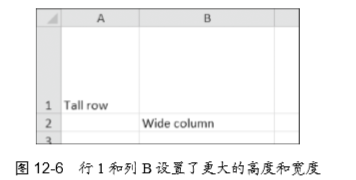一旦有了RowDimension对象，就可以设置它的高度。一旦有了ColumnDimension对 象，就可以设置它的宽度。行的高度可以设置为 0 到 409 之间的整数或浮点值。这 个值表示高度的点数。一点等于 1/72 英寸。默认的行高是 12.75。列宽可以设置为 0 到255 之间的整数或浮点数。这个值表示使用默认字体大小时（11 点），单元格可以显 示的字符数。默认的列宽是 8.43 个字符。列宽为零或行高为零，将使单元格隐藏。

合并和拆分单元格

利用 merge_cells()工作表方法，可以将一个矩形区域中的单元格合并为一个单 元格。在交互式环境中输入以下代码：

>>> import openpyxl
>>> wb = openpyxl.Workbook()
>>> sheet = wb.active
>>> sheet.merge_cells('A1:D3')
>>> sheet['A1'] = 'Twelve cells merged together.'
>>> sheet.merge_cells('C5:D5')
>>> sheet['C5'] = 'Two merged cells.'
>>> wb.save('merged.xlsx')

merge_cells()的参数是一个字符串，表示要合并的矩形区域左上角和右下角的 单元格：'A1:D3'将 12 个单元格合并为一个单元格。要设置这些合并后单元格的值， 只要设置这一组合并单元格左上角的单元格的值。 如果运行这段代码，merged.xlsx 看起来如图 12-7 所示。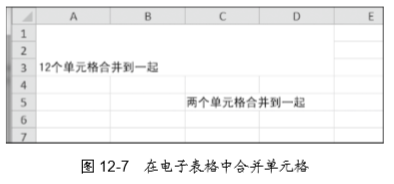要拆分单元格，就调用 unmerge_cells()工作表方法。在交互式环境中输入以下 代码：

>>> import openpyxl
>>> sheet = wb.get_active_sheet()
>>> sheet.unmerge_cells('A1:D3')
>>> sheet.unmerge_cells('C5:D5')
>>> wb.save('merged.xlsx') 

如果保存变更，然后查看这个电子表格，就会看到合并的单元格恢复成一些独 立的单元格。

冻结窗格

对于太大而不能一屏显示的电子表格，“冻结”顶部的几行或最左边的几列，是 很有帮助的。例如，冻结的列或行表头，就算用户滚动电子表格，也是始终可见的。 这称为“冻结窗格”。在 OpenPyXL 中，每个 Worksheet 对象都有一个 freeze_panes 属性，可以设置为一个 Cell 对象或一个单元格坐标的字符串。请注意，单元格上边的所 有行和左边的所有列都会冻结，但单元格所在的行和列不会冻结。 要解冻所有的单元格，就将 freeze_panes 设置为 None 或'A1'。表 12-3 展示了 freeze_panes 设定的一些例子，以及哪些行或列会冻结。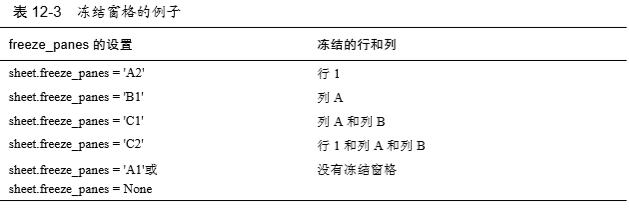确保你有来自 http://nostarch.com/automatestuff/的产品销售电子表格。然后在交 互式环境中输入以下代码：

>>> import openpyxl
>>> sheet = wb.active
>>> sheet.freeze_panes = 'A2'
>>> wb.save('freezeExample.xlsx')

如果将 freeze_panes 属性设置为'A2'，行 1 将永远可见，无论用户将电子表格滚 动到何处，如图 12-8 所示。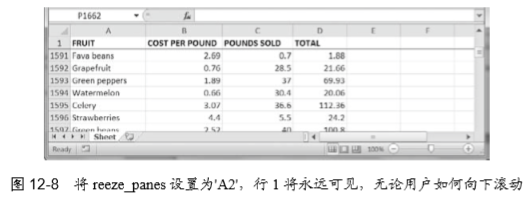图表

openpyxl 支持利用工作表中单元格的数据，创建条形图、折线图、散点图和饼 图。要创建图表，需要做下列事情：

1．从一个矩形区域选择的单元格，创建一个 Reference 对象。

2．通过传入 Reference 对象，创建一个 Series 对象。

3．创建一个 Chart 对象。

4．将 Series 对象添加到 Chart 对象。

5．可选地设置Chart对象的drawing.top、drawing.left、drawing.width和drawing.height 变量。

6．将 Chart 对象添加到 Worksheet 对象。

Reference 对象需要一些解释。Reference对象是通过调用 openpyxl.charts. Reference() 函数并传入 3 个参数创建的：

1．包含图表数据的 Worksheet 对象。

2．两个整数的元组，代表矩形选择区域的左上角单元格，该区域包含图表数 据：元组中第一个整数是行，第二个整数是列。请注意第一行是 1，不是 0。

3．两个整数的元组，代表矩形选择区域的右下角单元格，该区域包含图表数 据：元组中第一个整数是行，第二个整数是列。 图 12-9 展示了坐标参数的一些例子。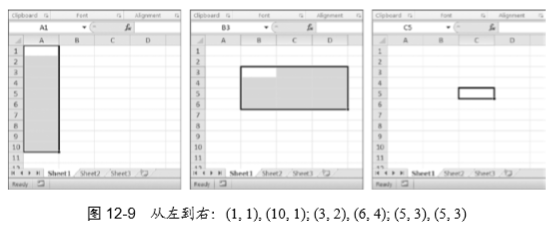在交互式环境中输入以下代码，创建一个条形图，将它添加到电子表格中：

>>> import openpyxl
>>> wb = openpyxl.Workbook()
>>> sheet = wb.get_active_sheet()
>>> for i in range(1, 11):   # create some data in column A
...    sheet['A' + str(i)] = i
...
>>> refObj = openpyxl.chart.Reference(sheet, (1, 1), (10, 1))
>>> seriesObj = openpyxl.chart.Series(refObj, title='First series')
>>> chartObj = openpyxl.charts.BarChart()
>>> chartObj.append(seriesObj)
>>> wb.save('sampleChart.xlsx') 

我们可以调用 openpyxl.charts.BarChart()，创建一个条形图。也可以调用 openpyxl.charts.LineChart()、openpyxl.charts.ScatterChart()和openpyxl.charts.PieChart()，创 建折线图、散点图和饼图。 遗憾的是，在 OpenPyXL 的当前版本中（2.1.4）， load_workbook()不会加载 Excel 文件中的图表。即使 Excel 文件包含图表，加载的 Workbook 对象也不会包含它们。 如果加载一个 Workbook 对象，然后马上保存到同样的.xlsx 文件名中，实际上就会 删除其中的图表。

展开全文• 下面小编就为大家分享一篇使用python获取csv文本的某或某数据的实例，具有很好的参考价值，希望对大家有所帮助。一起跟随小编过来看看吧
• 今天小编就为大家分享一篇python3读取excel文件只提取某些某些的值方法，具有很好的参考价值，希望对大家有所帮助。一起跟随小编过来看看吧
• 至于1D阵列而言，有的向量和列向量之间没有区别。他们完全一样。请看下面的例子，在这里我们得到了相同的结果，在所有情况下，这是不是在真正的线性代数(的理论意义)：In : wOut: array([0, 1, 2, 3, 4])...

当我试图计算w^T * x使用numpy的，对我来说是超级混乱以及。事实上，我自己无法实现。所以，这是NumPy中我们需要熟悉的几个陷阱之一。

至于1D阵列而言，有的行向量和列向量之间没有区别。他们完全一样。

请看下面的例子，在这里我们得到了相同的结果，在所有情况下，这是不是在真正的线性代数(的理论意义)：

In : w

Out: array([0, 1, 2, 3, 4])

In : x

Out: array([1, 2, 3, 4, 5])

In : np.dot(w, x)

Out: 40

In : np.dot(w.transpose(), x)

Out: 40

In : np.dot(w.transpose(), x.transpose())

Out: 40

In : np.dot(w, x.transpose())

Out: 40

有了这些信息，现在让我们尝试计算矢量的平方长度|w|^2。我们需要将w转换为2D数组。

In : wt = w[:, np.newaxis]

In : wt

Out:

array([,

,

,

,

])

现在，让我们来计算矢量w的平方长度(或平方幅度)：

In : np.dot(w, wt)

Out: array()

请注意，我们使用w，wt代替wt，w(如在理论线性代数)因为形状与使用np.dot(wt，w)不匹配。所以，我们有矢量的平方长度为。也许这是区分(numpy的解释)行列向量的方法之一吗？

最后，我有没有提到，我想通过实施w^T * x的方式？是的，我所做的：

In : wt

Out:

array([,

,

,

,

])

In : x

Out: array([1, 2, 3, 4, 5])

In : np.dot(x, wt)

Out: array()

所以，在与NumPy，操作数的顺序是相反的，如上述证明，相反的是，我们在理论上的线性代数研究。

展开全文• 前言数据的操作，在实际工作过程中应用非常广泛。由于不同人员、不同部门对数据结构的认识是不大相同的，尤其是从基层人员手里拿到的数据，更是五花八门，横七竖八。比如有这样一张成绩表：乍一看...

前言

数据的行转列操作，在实际工作过程中应用非常广泛。

由于不同人员、不同部门对数据结构的认识是不大相同的，尤其是从基层人员手里拿到的数据，更是五花八门，横七竖八。

比如有这样一张成绩表：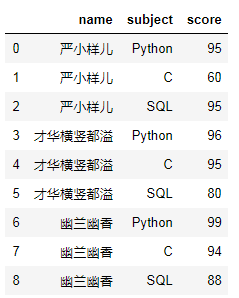乍一看，好像没毛病啊！！

然鹅鹅鹅，当需求：=简单计算一下每个人的总分吧！来临的时候。我脑海中不禁浮想起了：安排

# 遇事不要慌，先导个包吧
import pandas as pd
import numpy as np

# 造假数据
data = {'name':['严小样儿','严小样儿','严小样儿','才华横竖都溢','才华横竖都溢','才华横竖都溢','幽兰幽香','幽兰幽香','幽兰幽香'],
'subject':['Python','C','SQL','Python','C','SQL','Python','C','SQL'],
'score':[95,60,95,96,95,80,99,94,88]}

# 生成df
df = pd.DataFrame(data)
df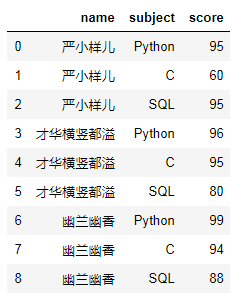使用pivot方法即可完成行转列哦~语法如下：

#df.pivot(index=None, columns=None, values=None)
df.pivot(index='name',columns='subject',values='score')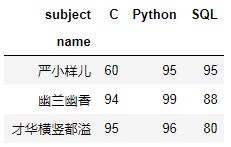不要高兴的太早，遇到重复值就麻烦了！少侠请看：

# 造含有重复值的假数据
data1 = {'name':['严小样儿','严小样儿','严小样儿','严小样儿','才华横竖都溢','才华横竖都溢','才华横竖都溢','幽兰幽香','幽兰幽香','幽兰幽香'],
'subject':['Python','Python','C','SQL','Python','C','SQL','Python','C','SQL'],
'score':[95,95,60,95,96,95,80,99,94,88]}

df1 = pd.DataFrame(data1)
df1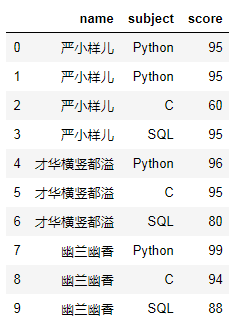df1.pivot(index='name',columns='subject',values='score')

# 一旦有重复值，就会报错。
ValueError: Index contains duplicate entries, cannot reshape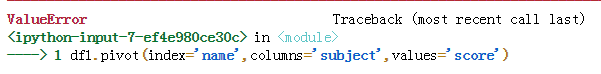别急别急，去个重不就可以了吗？！

df1.drop_duplicates().pivot(index='name',columns='subject',values='score')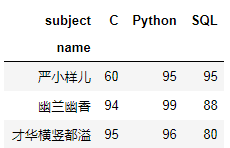方法二：数据透视表

# pivot_table(data, values=None, index=None, columns=None, aggfunc='mean')
pd.pivot_table(df1,index='name',columns='subject',values='score',aggfunc={'score':'max'})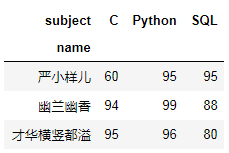聚合

刚刚说了，要求每个人的总分，其实使用透视表就可以完成。

不过，稍微动动脑筋哦。遇到重复值数据的话，只能使用下面的方法一，去重后的数据集，方法一，二都支持。

计算每个人的总分，语法如下：

# 重复数据集也可以
df_pivot = pd.pivot_table(df1,index='name',columns='subject',values='score',aggfunc={'score':'max'})
# 增加一个新列：Total
df_pivot['Total'] = df_pivot.apply(lambda x:np.sum(x),axis = 1)
df_pivot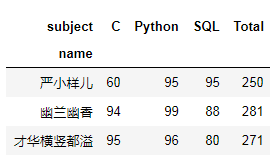方法二，必须是去重后的数据集，否则会出现计算错误。

# 使用去重数据集才可以
pd.pivot_table(df,index='name',values='score',aggfunc='sum')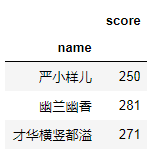# 使用join方法把总分列加进去。
total = pd.pivot_table(df,index='name',values='score',aggfunc='sum')
pd.pivot_table(df,index='name',columns='subject',values='score').join(total)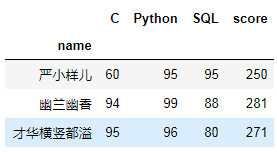--需求方：算是算出来了，可是，这个score看着怪怪的，能不能改成“总分”呢？

--严小样儿：我改（卑微）！安排~

total1 = pd.pivot_table(df,index='name',values='score',aggfunc='sum').rename({'score':'总分'},axis=1)
pd.pivot_table(df,index='name',columns='subject',values='score').join(total1)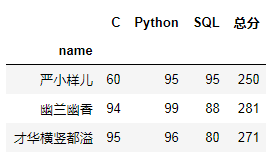行转列，就这样讲完了，大家赶快动手实践一下吧。那么，如何列转行呢？！

!

各位伙伴们好，詹帅本帅假期搭建了一个个人博客和小程序，汇集各种干货和资源，也方便大家阅读，感兴趣的小伙伴请移步小程序体验一下哦！（欢迎提建议）

推荐阅读

牛逼！Python常用数据类型的基本操作（长文系列第①篇）

牛逼！Python错误、异常和模块（长文系列第④篇）

展开全文机器学习 大数据 人工智能
• https://www.cnblogs.com/rrttp/p/8028421.html
• 您必须跟踪行和列号，因为xlrd Cell类似乎不存储：import sysimport xlrd_ordA = ord('A')def find_val_in_workbook(wbname, val):wb = xlrd.open_workbook(wbname)for sheet in wb.sheets():for rowi...
• 在处理电子表格时，可能经常需要在工作表中插入或删除行和列。因此，本文将介绍如何以编程方式处理...为了在Excel工作表中插入或删除行和列，我们将Aspose.Cells for Python via Java它是一个功能强大的电子表格处理Aaspose 文档管理
• 对于将数据发送到Excel，我将使用CSV而不是固定长度的文本格式；这样，如果您的浮点值中需要更重要的数字，那么输出的格式不会改变。此外，您只需在Excel中打开CSV文件；您不必导入它们。并且csv.writer为您处理所有...
• 所以我想创建一个新,以便新表可能如下所示： IndividualID Trip1 Trip2 Trip3 Trip4 Trip5 Trip6 Trip7 Trip8 Trip9 Chains 200100001 23 1 2 4 4 1 5 5 5 9 200100002 21 1 12 3 1 55 7 7 8 200100003 12 3 3 6 3...
• 关于行和列的参数解释 axis=0是跨, axis=1是跨 • axis=0： 0值表示沿着的方向或标签、索引值向下执行 • axis=1： 1值表示沿着的方向或标签值执行对应方法 可以看到当axis=0时，遍历的是每，或者说...numpy
• # ix——通过标签或者行号索引数据（基于lociloc 的混合） #取数据 print (data.loc[ 2 : 5 ]) #切片 print (data.iloc[ 2 : 5 ]) print (data.ix[ 2 : 5 ]) #取数据 print (data[[ "NDB_No" , "Vit...
• python中怎么用花括号定义一个54的数组花括号是字典，不是数组。python:定义函数,输入一个m维数组X一个整数n,输# Python3.6import numpy as npwhile True: x = input("输入一个m维数组,元素以空格分开：") try...
• import pandas as pd import numpy as np df=pd.DataFrame(np.arange(16).reshape(4,4),columns=[...对每一求和3.DataFrame中axis=1/axis=0的区别 1. 对每一求和 df.loc["sum"]=df.apply(lambda x:sum(x),axis=0)
• 直接上代码 import pandas as pd import numpy as np #生成行列格式数据 df=pd.DataFrame(np.random.randn(20).reshape(4,5),index=[['a','b','c','d']],columns=[10,20,30,40,50]) print(df) ...
• Worksheet 对象的 rows 属性 columns 属性得到的是一 Generator 对象，不能用中括号取索引。可先用列表推导式生成包含每一中所有单元格的元组的列表，在对列表取索引。Worksheet 的 rows 属性亦可用相同的方法...
• 需要取一个数据表里其中2列和前10的值： top10=pd_brand[['品牌','ASIN']][0:10]) #取列名称为品牌和ASIN的前10数据数据挖掘 机器学习
• 语法参数 DataFrame.set_index(i) 要修改的标签 DataFrame.rename(columns, index) columns：要修改的标签 index：要修改的标签 实例 import pandas as pd data = pd.read_excel('订单表.xlsx', ...机器学习 大数据 深度学习
• Sheet2,Sheet3 , 0表示第一个 TableNumber = open.sheets() # 获取行数 TotalNumberOfRows = TableNumber.nrows list_values = [] # 读取行数 range(start, start1) 某--某的信息 for x in range(start, ...excel
• 如题：python如何删除一 python如何删除一 list列表如何删除任意一或一 numpy如何删除一或一 利用numpy可以删除list的或者 删除一 import numpy as np list_data = [[1,2,3],[2,3,4],[4,5,6],[9,8...list 开发语言
• import numpy as np x = np.array([[1,2,3],[4,5,6]]) 输出x [[1 2 3] [4 5 6]] 转为向量 x=x.reshape(-1) 输出行向量 [1 2 3 4 5 6] 转为向量 x=x.reshape((-1,1)) 输出向量 [     ]
• 这篇文章主要介绍了Python+Xlwings 删除Excel的行和列的方法，帮助大家更好的理解使用python，感兴趣的朋友可以了解下一、需求：某公司管理的多个资管计划每天生成A表，业务人员需手工打开每个A表，将某些...
• python中基于numpy构建的矩阵，若需要获取其中一/的值，详细如下 import numpy as np a=np.array([[ 2, 7, 4, 2], [35, 9, 1, 5], [22, 12, 3, 2]]) print(a[:, ]) ## 第0 print(a[,:]) ## 第1 ...numpy矩阵
• 一、操作1.1 选择d = {'one' : pd.Series([1, 2, 3], index=['a', 'b', 'c']),'two' : pd.Series([1, 2, 3, 4], index=['a', 'b', 'c', 'd'])}df = pd.DataFrame(d)print (df ['one'])# 选择其中一进行显示，...
• 我们这里使用的是stack方法unstack方法。 stack：将转为。 unstack:将转为。（默认操作为最内层，可用分层级别的编号或名称对其他级别进行unstack） data = pd.DataFrame(np.arange(6).reshape((2,3...
• 首先需要导入第三方库 pip install openpyxl 这个库还是比较强大的。 接下来主要隐藏是 ...隐藏 sheet.column_dimensions.group(start_column,end_column,hidden=True) 值得注意的地方是的起始时数...
• 在使用Python处理表格时，pandas openpyxl是使用最多的两个库。现在我来简单记录一下这两个库在处理Excel表格时一些常用操作。 1、使用pandas操作excel表格 （1）读取指定单元格 # 导入pandas库，简写为pd ...数据挖掘 机器学习...